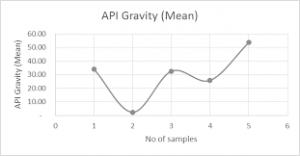## How to Calculate and Solve for Crude Oil Gravity, Oil Density and Water Density | The Calculator EncyclopediaThe image above represents crude oil gravity.

To compute for the crude oil gravity, two essential parameters are needed and these parameters are oil density (ρo) and water density (ρw).

The formula for calculating the crude oil gravity:

γo = ρo / ρw

Where;

γo = Crude Oil Gravity
ρo = Oil Density
ρw = Water Density

Let’s solve an example;
Find the crude oil gravity when the oil density is 28 and the water density is 46.

This implies that;

ρo = Oil Density = 28
ρw = Water Density = 46

γo = ρo / ρw
γo = 28 / 46
γo = 0.608

Therefore, the crude oil density is 0.608.

Calculating the Oil Density when the Crude Oil Gravity and the Water Density is Given.

ρo = γo x ρw

Where;

ρo = Oil Density
γo = Crude Oil Gravity
ρw = Water Density

Let’s solve an example;
Find the oil density when the crude oil gravity is 30 and the water density is 16.

This implies that;

γo = Crude Oil Gravity = 30
ρw = Water Density = 16

ρo = γo x ρw
ρo = 30 x 16
ρo = 480

Therefore, the oil density is 480.